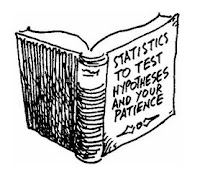## Pages

###ANOVA for Hypothesis Testing

It took me a lot of courage to write on the such a niche subject of Statistics. I swear, I used to quite petrified of statistical terms such as hypothesis testing, T Test, ANOVA, Chi-Square test etc., and still am little bit.

But when I tried to do it on SAS, actually performing these test is not that difficult. Let me try to help you understand the same.

The article has been written in continuation of previous article on T-test. In this article I am covering ANOVA in this one.

## Data for tests

Download the data, keep it at a location and assign the location to library "a" in SAS.

ANOVA is an abbreviation for Analysis of variance, but it is used to analyze the equality of mean (not variance), this way it seems to be a misnomer. However, its name is totally logical as in the background it calculates and compares the variance within group  and variance of groups together and hence establishes the equality of mean of groups.

ANOVA  provides a statistical test of to determine if the mean of several groups (even more than 2 at a time) are all equal or not. We can check whether the various samples are coming from the same sample or not. In T-test we can check equality of mean only of two samples at a time.

It is used if we want to study the effect of one or more qualitative variables (categorical) on a quantitative outcome variable. Such categorical variables are called Factors.

We have already explained the term "Hypothesis" in details in the previous part of this article covering T- test.

ANOVA test can be of various types depending on number of factors (categorical variable). Let's understand one way and two way types of ANOVA here.

### One Way ANOVA

Let us understand it by example. In the data, there are three variables :

1.  Sales value of  XYZ category
2.  Media Spend as  High, Medium and Low ( based on how many \$ were spent on promotion)
3.  Festive Season Flag ( Yes or No)

Now suppose, we have a hypothesis that Sales is independent of media spend.

So the null hypothesis is equality of means:

H0 :    Mean sales with High, Medium, and Low media spend are same
H1 :    Mean sales of at least one category is significantly different

Let's perform the test in SAS.

Proc ANOVA data = a.sales;
Class Media_Spend  ;
Model Sales = Media_Spend   ;
Run;
Quit;

As the p value is < 0.01, we can definitely reject the null and we can say that the sales  among groups is significantly different and hence we can conclude that Media spend affects sales significantly.

Enjoy reading our other articles and stay tuned with ...

Kindly do provide your feedback in the 'Comments' Section and share as much as possible.